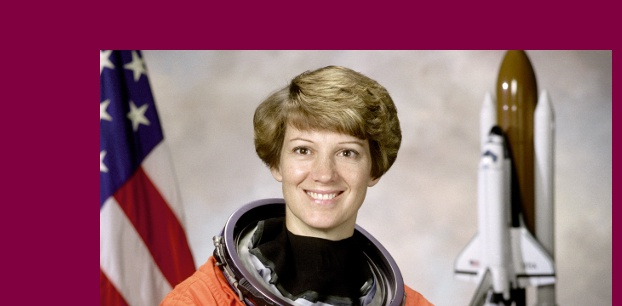# note.nkmk.me

Posted: 2019-05-14 / Tags: Python, Pillow, Image Processing

If you want to resize an image but do not want to change the aspect ratio or trim it, you can adjust the size by adding padding to the top, bottom, left, and right of the image.

You can pad an image by using `new()` and `paste()` of the Python image processing library Pillow (PIL).

See the following article for the installation and basic usage of Pillow (PIL).

See the following article about trimming.

Import `Image` from `PIL` and load the original image.

``````from PIL import Image

im = Image.open('data/src/astronaut_rect.bmp')
``````Generate a solid image with `new()`, and paste the original image with `paste()`.

Define the following function.

Specify the width of the margin to `top`,`right`, `bottom`,`left`, and the background color `(R, G, B)` (maximum value is `255`) to `color`.

``````def add_margin(pil_img, top, right, bottom, left, color):
width, height = pil_img.size
new_width = width + right + left
new_height = height + top + bottom
result = Image.new(pil_img.mode, (new_width, new_height), color)
result.paste(pil_img, (left, top))
return result
``````
source: imagelib.py

Usage example:

``````im_new = add_margin(im, 50, 10, 0, 100, (128, 0, 64))
``````Add padding to the short side to make it square while maintaining the aspect ratio of the rectangular image.

Define the following function.

``````def expand2square(pil_img, background_color):
width, height = pil_img.size
if width == height:
return pil_img
elif width > height:
result = Image.new(pil_img.mode, (width, width), background_color)
result.paste(pil_img, (0, (width - height) // 2))
return result
else:
result = Image.new(pil_img.mode, (height, height), background_color)
result.paste(pil_img, ((height - width) // 2, 0))
return result
``````
source: imagelib.py

Usage example:

``````im_new = expand2square(im, (0, 0, 0))
im_new.save('data/dst/astronaut_expand_square.jpg', quality=95)
``````When you want to make a square of a certain size, such as making a thumbnail, add padding and then `resize()`.

``````im_new = expand2square(im, (0, 0, 0)).resize((150, 150))
``````